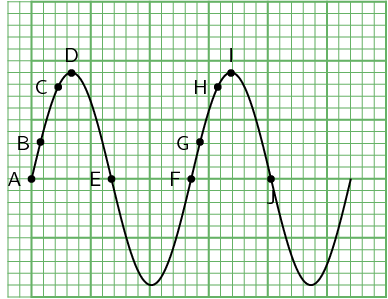Home Practice
For learners and parents For teachers and schools
Textbooks
Full catalogue
Pricing SupportLog in

We think you are located in United States. Is this correct?

# 8.5 Points in phase

## Points in phase

Fill in the table by measuring the distance between the indicated points.Points Distance (cm) A to F B to G C to H D to I E to J

What do you find?

In the activity the distance between the indicated points was equal. These points are then said to be in phase. Two points in phase are separate by a whole ($$\text{1}$$; $$\text{2}$$; $$\text{3}$$; …) number multiple of whole wave cycles or wavelengths. The points in phase do not have to be crests or troughs, but they must be separated by a complete number of wavelengths.

We then have an alternate definition of the wavelength as the distance between any two adjacent points which are in phase.

Wavelength of wave

The wavelength of a wave is the distance between any two adjacent points that are in phase.

Points that are not in phase, those that are not separated by a complete number of wavelengths, are called out of phase. Examples of points like these would be A and C, or D and E, or B and H in the Activity.

temp text